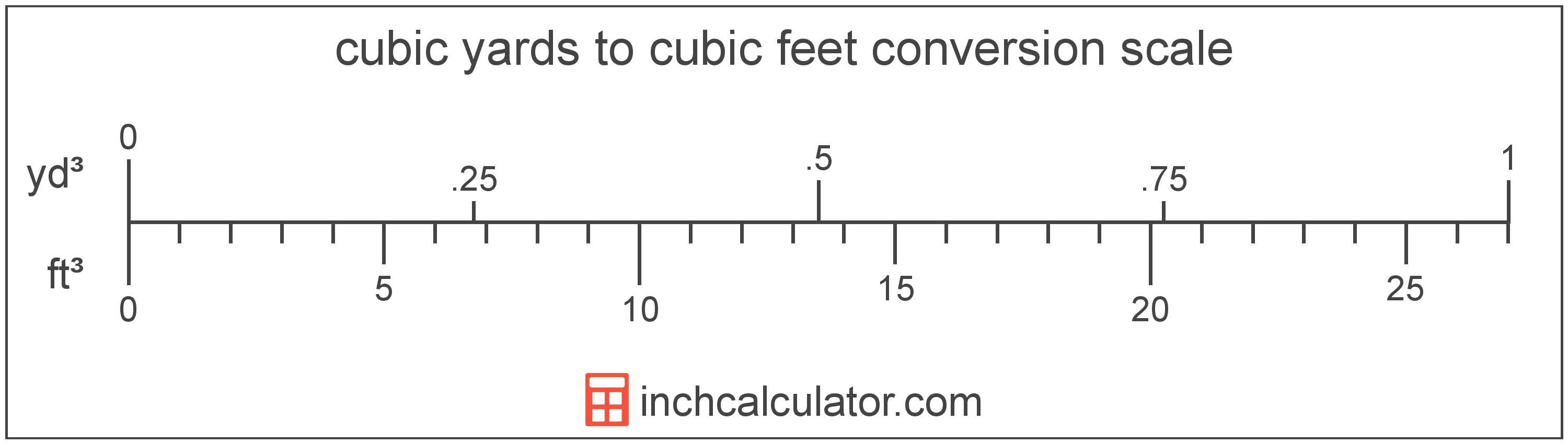# Cubic Yards to Cubic Feet Conversion

Enter the volume in cubic yards below to get the value converted to cubic feet.

(find cubic yards)
Results in Cubic Feet:1 yd³ = 27 ft³

## How to Convert Cubic Yards to Cubic Feet

To convert a cubic yard measurement to a cubic foot measurement, multiply the volume by the conversion ratio.

Since one cubic yard is equal to 27 cubic feet, you can use this simple formula to convert:

cubic feet = cubic yards × 27

The volume in cubic feet is equal to the cubic yards multiplied by 27.

For example, here's how to convert 5 cubic yards to cubic feet using the formula above.
5 yd³ = (5 × 27) = 135.000002 ft³### How Many Cubic Feet are in a Cubic Yard?

There are 27 cubic feet in a cubic yard, which is why we use this value in the formula above.

1 yd³ = 27 ft³

## Cubic Yards

The cubic yard is a unit of volume that is equal to the space consumed by a cube with each edge measuring one yard.

The cubic yard is a US customary and imperial unit of volume. A cubic yard is sometimes also referred to as a cubic yd. Cubic yards can be abbreviated as yd³, and are also sometimes abbreviated as cu yard, cu yd, or CY. For example, 1 cubic yard can be written as 1 yd³, 1 cu yard, 1 cu yd, or 1 CY.

You might also be interested in our cubic yards calculator.

## Cubic Feet

The cubic foot is a unit of volume that is equal to the space consumed by a cube having sides one foot on each edge.

The cubic foot is a US customary and imperial unit of volume. A cubic foot is sometimes also referred to as a cubic ft. Cubic feet can be abbreviated as ft³, and are also sometimes abbreviated as cu foot, cu ft, or CF. For example, 1 cubic foot can be written as 1 ft³, 1 cu foot, 1 cu ft, or 1 CF.

Try our cubic footage calculator to calculate the volume of a space.

## Cubic Yard to Cubic Foot Conversion Table

Cubic yard measurements converted to cubic feet
Cubic Yards Cubic Feet
1 yd³ 27 ft³
2 yd³ 54 ft³
3 yd³ 81 ft³
4 yd³ 108 ft³
5 yd³ 135 ft³
6 yd³ 162 ft³
7 yd³ 189 ft³
8 yd³ 216 ft³
9 yd³ 243 ft³
10 yd³ 270 ft³
11 yd³ 297 ft³
12 yd³ 324 ft³
13 yd³ 351 ft³
14 yd³ 378 ft³
15 yd³ 405 ft³
16 yd³ 432 ft³
17 yd³ 459 ft³
18 yd³ 486 ft³
19 yd³ 513 ft³
20 yd³ 540 ft³
21 yd³ 567 ft³
22 yd³ 594 ft³
23 yd³ 621 ft³
24 yd³ 648 ft³
25 yd³ 675 ft³
26 yd³ 702 ft³
27 yd³ 729 ft³
28 yd³ 756 ft³
29 yd³ 783 ft³
30 yd³ 810 ft³
31 yd³ 837 ft³
32 yd³ 864 ft³
33 yd³ 891 ft³
34 yd³ 918 ft³
35 yd³ 945 ft³
36 yd³ 972 ft³
37 yd³ 999 ft³
38 yd³ 1,026 ft³
39 yd³ 1,053 ft³
40 yd³ 1,080 ft³